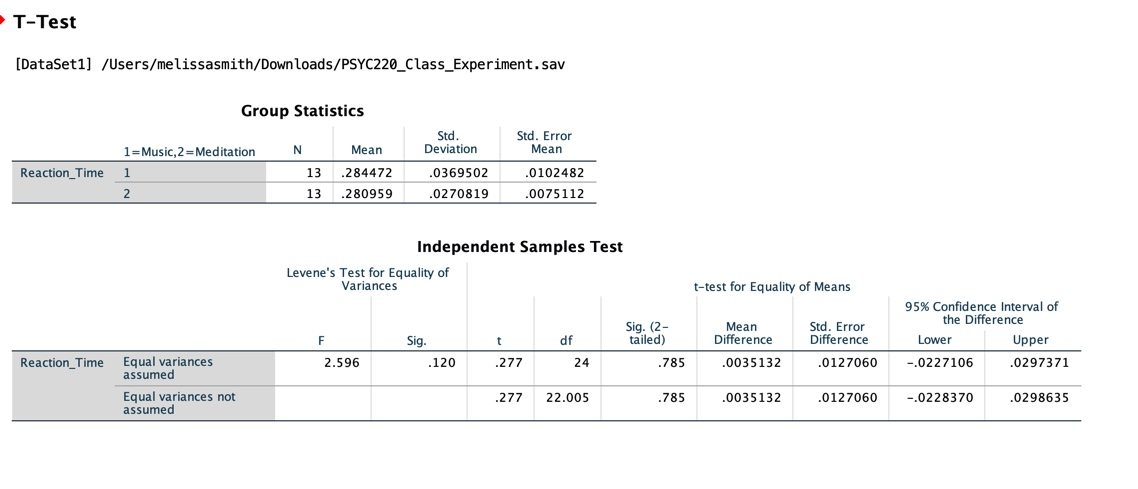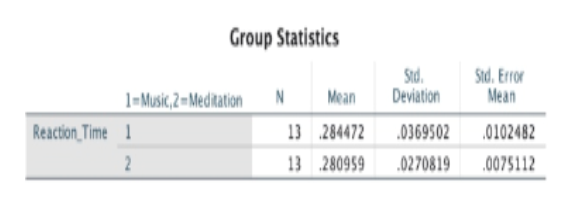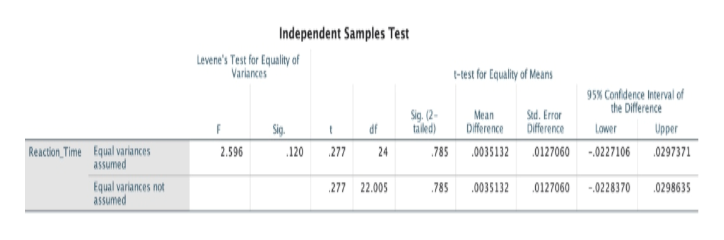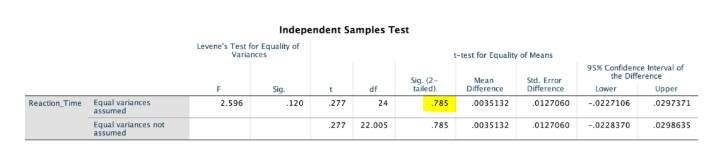# T-Test[DataSet1] /Users/melissasmith/Down loads/PSYC220_Class_Experiment.savGroup StatisticsStd.DeviationStd. ErrorMeanNMean1 - Music, 2 Med itationReaction_Time 113284472.0369502010248228095902708192130075112Independent Samples TestLevene's Test for Equality ofVariancest-test for Equality of Means95% Confidence Interval ofthe DifferenceSig. (2-tailed)Std. ErrorDifferenceMeanDifferencedfLowerUpperFSig.Reaction_Time Equal variancesassumed-.02271062.596120.27724.785.003513201270600297371Equal variances notassumed277.003513222.0057850127060-.0228370.0298635

Question
45 views

See attached photo of data

Using 0.5 as you alpha level for the levines test, the "sig" value for Levenes test is ______.

Under the section titled t test for equality of mean what are the values for t, df, and Sig 2 tailed? Is the Difference between groups significant? ie <0.05?________________________________________________________

Briefly describe the out come of the experiment. was the difference between music and meditation groups significant? What does that mean in terms of the effect of meditation on reaction time? __________________________________________________________help_outlineImage TranscriptioncloseT-Test [DataSet1] /Users/melissasmith/Down loads/PSYC220_Class_Experiment.sav Group Statistics Std. Deviation Std. Error Mean N Mean 1 - Music, 2 Med itation Reaction_Time 1 13 284472 .0369502 0102482 280959 0270819 2 13 0075112 Independent Samples Test Levene's Test for Equality of Variances t-test for Equality of Means 95% Confidence Interval of the Difference Sig. (2- tailed) Std. Error Difference Mean Difference df Lower Upper F Sig. Reaction_Time Equal variances assumed -.0227106 2.596 120 .277 24 .785 .0035132 0127060 0297371 Equal variances not assumed 277 .0035132 22.005 785 0127060 -.0228370 .0298635 fullscreen
check_circle

star
star
star
star
star
1 Rating
Step 1

All the answers are based on the output of test performed by you. This will help you read the output and glean insights from it.

From the Group Statistics table below, we can see that there are 13 subjects in each of the groups namely Music group and Meditation group.

Average reaction time of Music group is 0.284472 and Average reactiontime of Meditation group is 0.280959. If we only look at the means, the difference does not seem significant but further analysis of other parameters tells another story.help_outlineImage TranscriptioncloseGroup Statistics Std. Deviation Std. Error Mean Mean 1-Music,2=MedRation Reaction,Time 1 13 284472 0369502 0102482 2 13 280959 0270819 0075112 fullscreen
Step 2

This table has a lot of data but we will not need all of it for our analysis. There are 2 rows - Equal Variances assumed and unequal variances assumed. We will use just one row depending on the value given in Sig column (2nd column). If the value here is greater than 0.05, we wil read from the top row ( Equal Variances assumed) assuming that there is no significant difference between the variability of the Music and Meditation groups. If the value under Sig column is less than 0.05. we will conclude that there is statistically significant variability etween the two groups and in this case, we read from the bottom row.

Value under Sig column is 0.12 which is greater than 0.05. We will thus read from top row for our analysis.help_outlineImage TranscriptioncloseIndependent Samples Test Levene's Test for Equality of Variances -Hest for Equality of Means 95% Confidence Interval of the Difference Sig.(2 taed) Sad. Error Oifference Mean Diference Sig df Lower Upper Reaction Time Equal variances assumed 2.596 277 24 785 0035132 0127060 -0227106 0297371 120 Equal variances not assumed 277 22.005 785 0035132 0127060 0228370 0298635 fullscreen
Step 3

In top row, we look at the Sig(2-tailed) column value which tells us if there isstatistically  significant difference between the means of two groups. If the value is greater than 0.05, we conclude that there is no statistically significant difference between the means of the two groups. If the valu...help_outlineImage TranscriptioncloseIndependent Samples Test Levene's Test for Equality of Variances t-test for Equality of Means 95 % Confidence Interval of the Difference Sig. (2 tailed) Mean Difference Std. Error Difference Sig. df Lower Upper t Equal variances assumed .0035132 Reaction Time 2.596 120 277 24 785 0127060 -0227106 0297371 Equal variances not assumed 22.005 277 785 0035132 0127060 -.0228370 0298635 fullscreen

### Want to see the full answer?

See Solution

#### Want to see this answer and more?

Solutions are written by subject experts who are available 24/7. Questions are typically answered within 1 hour.*

See Solution
*Response times may vary by subject and question.
Tagged in

### Hypothesis Testing